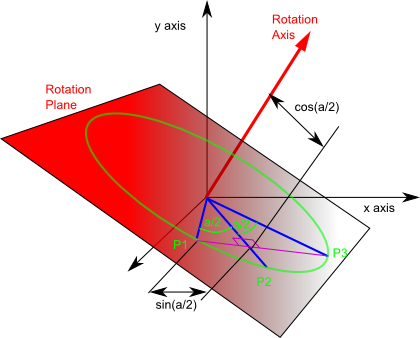Maths - Quaternion Notations - As a quantity similar to axis-angle

It is quite difficult to give a physical meaning to a quaternion, it is just a quantity which represents a rotation. If you need a physical meaning then this is probably the best way to think of it:

q = cos(a/2) + i ( x * sin(a/2)) + j (y * sin(a/2)) + k ( z * sin(a/2))

where:

• a=angle of rotation.
• x,y,z = vector representing axis of rotation.

So it is closely related to the axis angle representation of rotations.

In the following diagram:

• Rotation Axis: is a line we are rotating around, that is, during the rotation points maintain the same distance from the rotation axis.
• Rotation Plane: During the rotation points in the plain remain in the plane and other points remain the same distance from the plane.

Note: we are talking about rotations about the origin so both the rotation axis and the rotation plane go through the origin.Imagine a point P1 which is a unit distance from the origin, we will be rotating it through an angle (a) to P3 through midpoint P2.So the point P1 is transformed to P3, if it travels in a straight line it passes through a point cos(a/2) from the rotation axis and sin(a/2) from both P1 and P3.